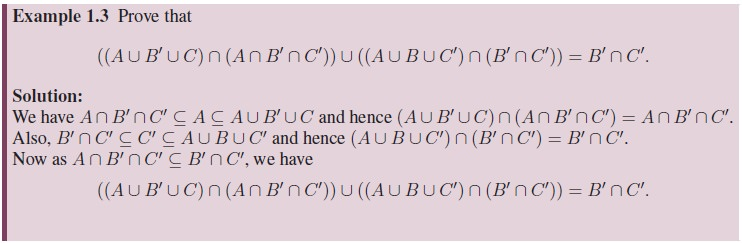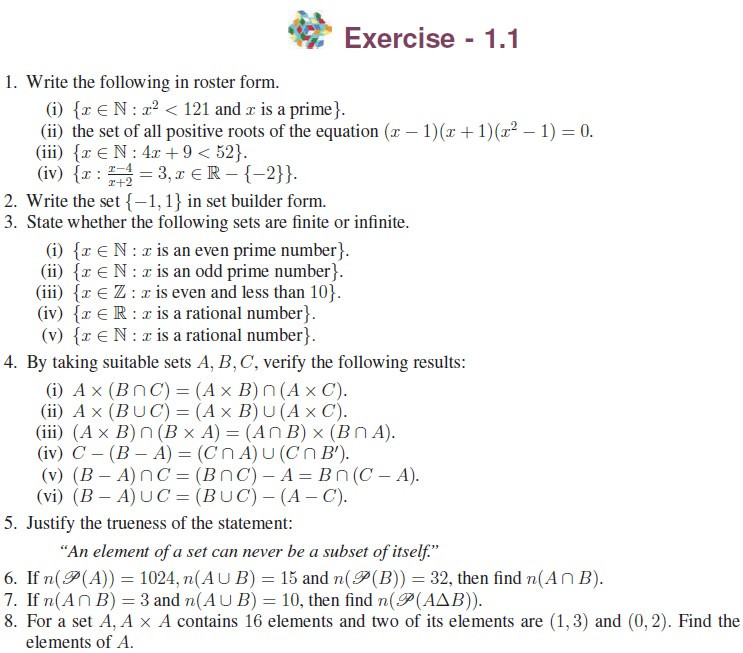Home | | Maths 11th std | Cartesian Product

# Cartesian Product

In particular, Cartesian product of two sets is a set of ordered pairs, while the Cartesian product of three sets is a set of ordered triplets. Precisely, let A, B and C be three non-empty sets.

Cartesian Product

We know that the Cartesian product of sets is nothing but a set of ordered elements. In particular, Cartesian product of two sets is a set of ordered pairs, while the Cartesian product of three sets is a set of ordered triplets. Precisely, let A, B and C be three non-empty sets. Then the Cartesian product of A with B is denoted by A × B. It is defined byHere A × B is a subset of R × R. The number of elements in A × B is the product of the number of elements in A and the number of elements in B, that is, n(A × B) = n(A)n(B), if A and B are finite. Further n(A × B × C) = n(A)n(B)n(C), if A, B and C are finite.

It is easy to see that the following are the subsets of R × R.Tags : Definition, Formula, Solved Example Problems, Exercise | Mathematics , 11th Mathematics : UNIT 1 : Sets, Relations and Functions
Study Material, Lecturing Notes, Assignment, Reference, Wiki description explanation, brief detail
11th Mathematics : UNIT 1 : Sets, Relations and Functions : Cartesian Product | Definition, Formula, Solved Example Problems, Exercise | Mathematics